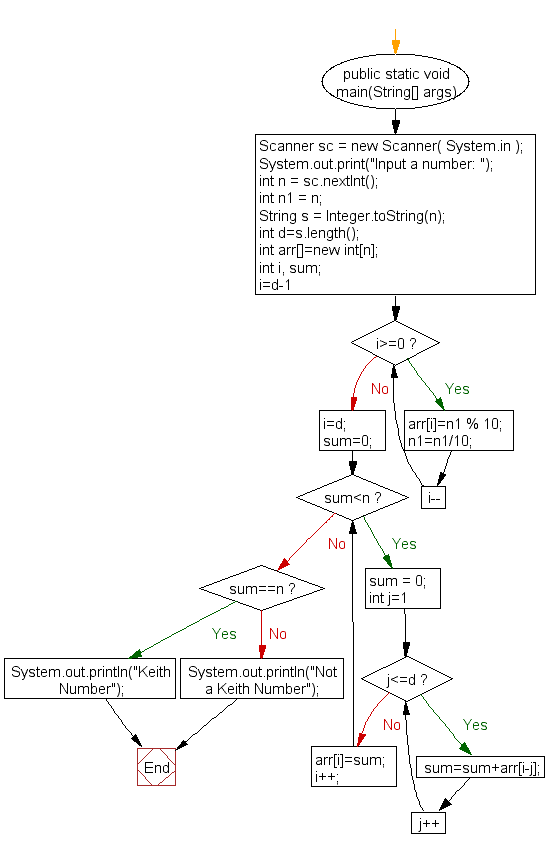﻿ Java exercises: Check whether a number is a Keith Number or not - w3resource# Java Exercises: Check whether a number is a Keith Number or not

## Java Numbers: Exercise-26 with Solution

Write a Program in Java to check whether a number is a Keith Number or not.

In recreational mathematics, a Keith number or repfigit number (short for repetitive Fibonacci-like digit) is a number in the following integer sequence:
14, 19, 28, 47, 61, 75, 197, 742, 1104, 1537, 2208, 2580, 3684, 4788, 7385, 7647, 7909, 31331, 34285, 34348, 55604, 62662, 86935, 93993, 120284, 129106, 147640, 156146, 174680, 183186, 298320, 355419, 694280, 925993,

Test Data
Input a number: 75

Sample Solution:

Java Code:

``````import java.util.Scanner;
public class Example26  {

public static void main(String[] args) {

Scanner sc = new Scanner( System.in );
System.out.print("Input a number: ");
int n = sc.nextInt();
int n1 = n;
String s = Integer.toString(n);
int d=s.length();
int arr[]=new int[n];
int i, sum;
for(i=d-1; i>=0; i--)
{
arr[i]=n1 % 10;
n1=n1/10;

}

i=d; sum=0;
while(sum<n)
{
sum = 0;
for(int j=1; j<=d; j++)
{
sum=sum+arr[i-j];
}
arr[i]=sum;
i++;
}

if(sum==n)
System.out.println("Keith Number");
else
System.out.println("Not a Keith Number");
}
}
```
```

Sample Output:

```Input a number: 75
Keith Number
```

Flowchart:Java Code Editor:

What is the difficulty level of this exercise?

﻿

## Java: Tips of the Day

Array vs ArrayLists:

The main difference between these two is that an Array is of fixed size so once you have created an Array you cannot change it but the ArrayList is not of fixed size. You can create instances of ArrayLists without specifying its size. So if you create such instances of an ArrayList without specifying its size Java will create an instance of an ArrayList of default size.

Once an ArrayList is full it re-sizes itself. In fact, an ArrayList is internally supported by an array. So when an ArrayList is resized it will slow down its performance a bit as the contents of the old Array must be copied to a new Array.

At the same time, it's compulsory to specify the size of an Array directly or indirectly while creating it. And also Arrays can store both primitives and objects while ArrayLists only can store objects.

Ref: https://bit.ly/3o8L2KH